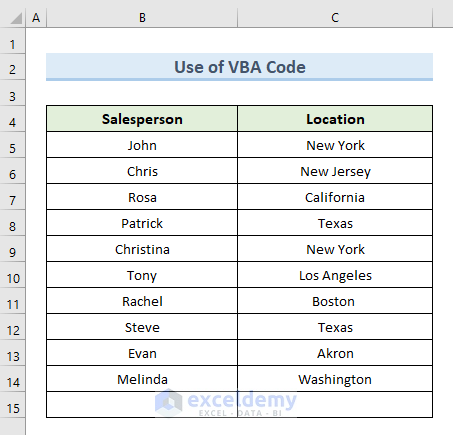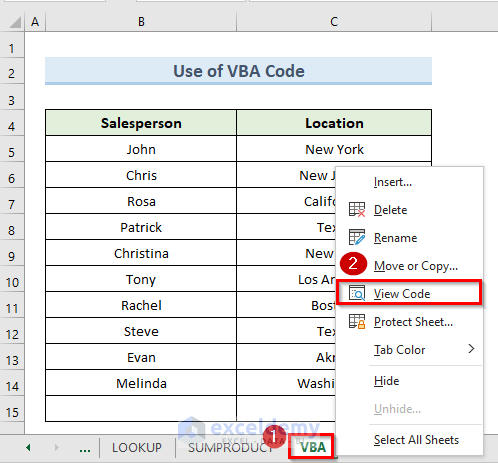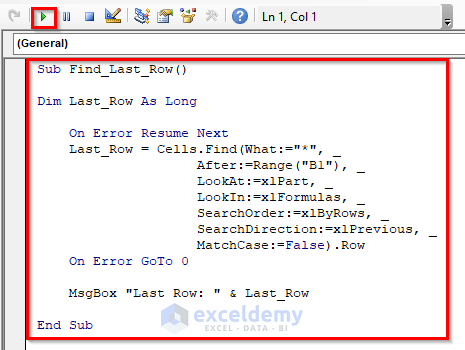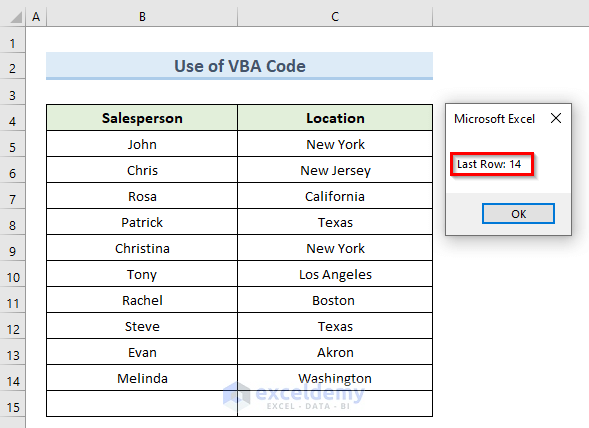# How to Use Excel Formula to Find Last Row Number with Data (2 Ways)

Get FREE Advanced Excel Exercises with Solutions!

In this tutorial, we will demonstrate how to use an excel formula to find the last row number with data. While working in Microsoft Excel we might need to know the last row number from a data range. To find the last row number with data we will use different formulas throughout this article. If your working process demands to create a dynamic data range then you have to find the last row number of your data range. In that case, this article will help you a lot.

## 2 Ways to Use Excel Formula to Find Last Row Number with Data

In this article, we will use an excel formula to find the last row number with data for two cases. The last row of our dataset can be either blank or non-blank. The output of the last row number with data will not be the same for both cases. So, we will use different approaches for the above cases to find the last row number with data.

We have a dataset of salespeople and their location in the following screenshot. Throughout all the methods of this article, we will use the same dataset to find the last row number with data.

### 1. Excel Formula to Find Non-Blank Last Row Number with Data

In the first section, we will discuss the excel formulas to find last row numbers with data for non-blank cells. The formulas that we will discuss in the 3 methods of this section will not be applicable if one or multiple last rows are blank in a data range.

#### 1.1 Formula with ROW and ROWS Functions to Find Last Row Number with Data in Excel

First and foremost, we will use a combination of the ROW and ROWS functions to find the last row number with data in excel.

The Excel ROW function returns the row number from the active worksheet.

The ROWS function in Excel returns the number of rows in a specified reference.

We will find the last row number of the following dataset in cell E5.Let’s take a look at the steps to do this.

STEPS:

• Firstly, select cell E5.
• Secondly, insert the following formula in that cell:
`=ROW(B5:C15) + ROWS(B5:C15)-1`• Press Enter.
• The above action returns the row number of the last row from the data range in cell E5. We can see that the number of the last row is 15.Read More: How to Find Last Row with a Specific Value in Excel (6 Methods)

#### 1.2 Combine MIN, ROW, and ROWS Functions to Find Last Row Number with Data in Excel

In this method to find the last row number with data in excel, we will combine MIN, ROW, and ROWS functions.

The MIN function in Excel returns the data’s smallest number value from a data range.

In the following dataset, we will find the last row number of the dataset.Let’s see the steps to perform this action.

STEPS:

• First, select cell C5.
• Next, insert the following formula in that cell:
`=MIN(ROW(B5:C15))+ROWS(B5:C15)-1`
• Then, press Enter.
• Finally, the above command returns the number of the last row in cell E5.🔎 How Does the Formula Work?

• ROW(B5:C15))+ROWS(B5:C15)-1: This part returns the array of row numbers from the last row which is row number 15.
• MIN(ROW(B5:C15))+ROWS(B5:C15)-1: Returns the minimum row number in cell E5 which is row number 15.

Read More: Excel Find Last Occurrence of Character in String (6 Methods)

#### 1.3 Find Last Row Number with Data Using Excel Formula with ROW, INDEX, and ROWS Functions

Another method to use excel formulas to find the last row number with data is to use a combination of ROW, INDEX, and ROWS functions.

In Microsoft Excel, the INDEX function returns the value at a certain position in a range or array.

We will find the number of the last row from the following dataset.Let’s see the steps to perform this action.

STEPS:

• In the beginning, select cell E5.
• Next, input the following formula in that cell:
`=ROW(INDEX(B5:C15,1,1))+ROWS(B5:C15)-1`
• After that, press Enter.
• Finally, we get the last row number of our data range in cell E5 which is 15.🔎 How Does the Formula Work?

• INDEX(B5:C15,1,1): This part creates an array of the data range (B5:C15).
• ROWS(B5:C15)-1: This part subtracts 1 from the total row numbers.
• ROW(INDEX(B5:C15,1,1))+ROWS(B5:C15)-1: Returns the minimum row number in cell E5 which is row number 15.

Read More: Excel Find Last Column With Data (4 Quick Ways)

### 2. Find Both Blank and Non-Blank Last Row Number with Data in Excel

If one or multiple last rows from or data range are blank the above formulas will not work to find the last row number with data in excel. Because the following formula doesn’t look for whether the last row is blank or not. It just returns the number of the last row from the given data range. In this section, we will discuss the formulas which are applicable for both blank and non-blank rows.

#### 2.1. Insert MAX Formula to Find Last Row Number with Data in Excel

Now, to find the last row number from a dataset that has one or multiple blank rows we will use the MAX function.

The excel MAX function provides the greatest value in a specified set of data.

In the following dataset, we will find the last row number in cell E5 with the help of the excel MAX function. If we notice we will see that the last row of the dataset doesn’t contain any value.Let’s see the steps to do this method.

STEPS:

• Firstly, select cell E5.
• Secondly, input the following formula in that cell:
`=MAX((B:B<>"")*(ROW(B:B)))`
• Then, press Enter.
• Lastly, we get the last row number in cell E5 which is 14. It excludes the last row of our data range which is blank.Read More: How to Find Last Non Blank Cell in Row in Excel (5 Methods)

#### 2.2. Combine MATCH and REPT Functions to Find Last Row Number with Data in Excel

Using the combination of MATCH and REPT functions is another way to find the last row number with data in excel.

The MATCH function in excel searches a range of cells for a specified item. Then it returns the item’s relative location in the range.

The REPT function in excel repeats specific text a given number of times. We can use the REPT function to fill a cell with multiple instances of a text string.

In the following dataset, we will find the last row number with data in cell E5.Let’s see the steps to perform this action.

STEPS:

• First, select cell E5.
• Then, insert the following formula in that cell:
`=MATCH(REPT("z",50),B:B)`
• Press Enter.
• Finally, in cell E5 we get the number of the last row with data in our dataset.🔎 How Does the Formula Work?

• REPT(“z”,50): This part repeats the text ‘z50 times.
• MATCH(REPT(“z”,50),B:B): In this part, the MATCH function looks in column B for our 50-character text string of ‘z’. The formula returns the location of the last non-blank cell since it cannot find it.

Read More: Find Last Value in Column Greater than Zero in Excel (2 Easy Formulas)

#### 2.3 Use Excel LOOKUP Formula to Find Last Row Number with Data

We can also use the LOOKUP formula to find the last row number with data.

The LOOKUP function belongs to the Excel Lookup and Reference functions. The LOOKUP function returns the comparable value from another one-row or one-column range after performing an approximate match lookup.

In the following dataset, the last row is blank. We will find the row number of the last row with data in cell E5.Let’s see the steps to use the LOOKUP function.

STEPS:

• In the beginning, select cell E5.
• Next, enter the following formula in that cell:
`=LOOKUP(2,1/(B:B<>""),ROW(B:B))`
• Press, Enter.
• Finally, we can see the last row number of our data range in cell E5 which is 14.Read More: How to Find Lowest 3 Values in Excel (5 Easy Methods)

#### 2.4 Identify Last Row Number with Data in Excel Using SUMPRODUCT Function

In this method, we will use the SUMPRODUCT function to identify the last row number with data in excel.

The SUMPRODUCT function in Excel returns the sum of matching ranges or arrays’ products.

The last row in the following dataset is blank. In cell E5, we’ll find the row number of the last row with data.Let’s see the steps to do this method.

STEPS:

• First, select cell E5.
• Then, write down the following formula in that cell:
`=SUMPRODUCT(MAX((C5:C15<>"")*ROW(C5:C15)))`
• Press Enter if you are using ‘Microsoft Office 365’ otherwise you have to press Ctrl + Shift + Enter to run an array.
• In the end, we get the last row number with data in cell E5.🔎 How Does the Formula Work?

• ROW(C5:C15): This part returns the row number for each cell in range (C5:C15).
• MAX((C5:C15<>””): This part returns the highest number from the array of row numbers.
• SUMPRODUCT(MAX((C5:C15<>””)*ROW(C5:C15))): The SUMPRODUCT function is used to calculate the above two arrays and return a value in the selected cell.

Read More: How to Find Last Cell with Value in Column in Excel

#### 2.5 Detect Last Row Number with Data in Excel with VBA code

We can easily use VBA (Visual Basic for Applications) code to detect the last row number with data in excel. In the following dataset, the last row is blank. We will use a VBA code to find the last row number that is not blank.

##Let’s see the steps to apply a VBA Code to find the last row number with data.

STEPS:

• Firstly, right-click on the sheet name of the active sheet.
• Secondly, click on the option ‘View Code’.

##• A new blank VBA module will appear.
• Thirdly, write the following code in the blank module:
``````Sub Find_Last_Row()
Dim Last_Row As Long
On Error Resume Next
Last_Row = Cells.Find(What:="*", After:=Range("B1"), _
LookAt:=xlPart, LookIn:=xlFormulas, _
SearchOrder:=xlByRows, SearchDirection:=xlPrevious, _
MatchCase:=False).Row
On Error GoTo 0
MsgBox "Last Row: " & Last_Row
End Sub``````
• Then, click on the Run or press the F5 key to run the code.

##• Lastly, the above command shows a message box. In the message box, we can see the last row number with data which is 14.

##Read More: Find the Last Cell With Value in Row in Excel (6 Methods)

## Conclusion

In conclusion, this tutorial will demonstrate how to use excel formulas to find the last row number with data. To put your skills to the test, use the practice worksheet that comes with this article. Please leave a comment below if you have any questions. Our team will try our best to reply to you as soon as possible. In the future, keep an eye out for more innovative Microsoft Excel solutions.

## Related ArticlesMukesh Dipto

Hi there! I am Mukesh Dipto. I graduated with a bachelor's degree in engineering. Currently, I am working as a technical content writer in ExcelDemy. You will find all my articles on Microsoft Excel on this site. Outside of the workplace, my hobbies and interests include sports and meeting new people. I also enjoy sports. My favorite sports are football (to watch and play) and Badminton (play).

We will be happy to hear your thoughtsAdvanced Excel Exercises with Solutions PDF